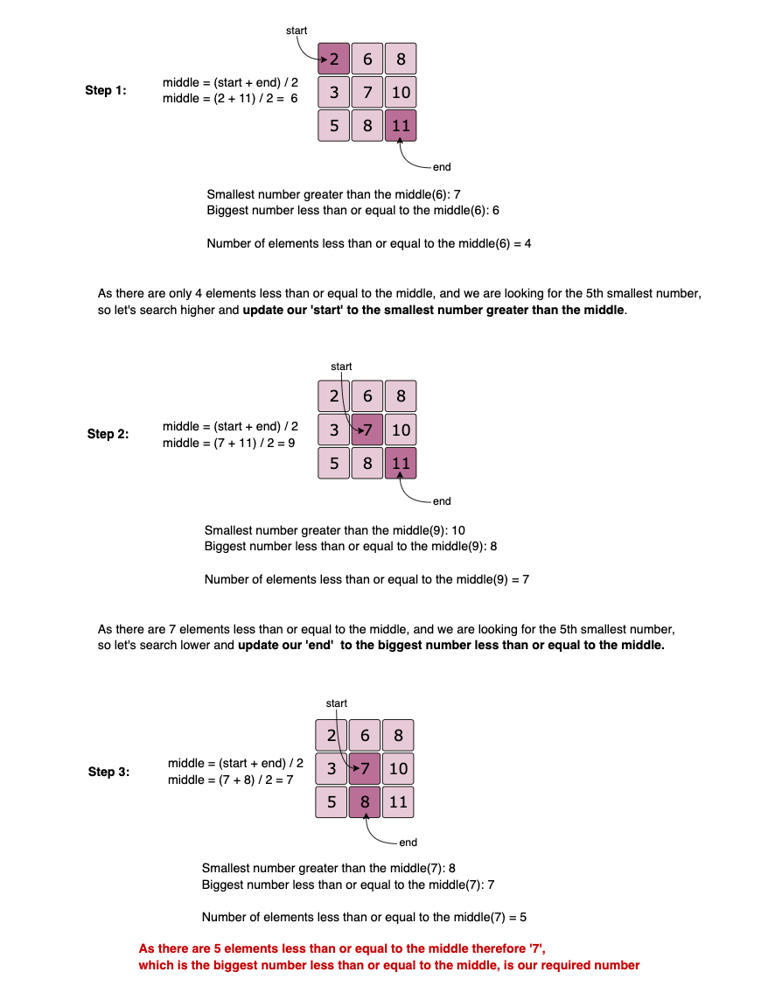0378. Kth Smallest Element in a Sorted Matrix

# 378. Kth Smallest Element in a Sorted Matrix#

## 题目 #

Given a n x n matrix where each of the rows and columns are sorted in ascending order, find the kth smallest element in the matrix.

Note that it is the kth smallest element in the sorted order, not the kth distinct element.

Example:

``````matrix = [
[ 1,  5,  9],
[10, 11, 13],
[12, 13, 15]
],
k = 8,

return 13.
``````

Note: You may assume k is always valid, 1 ≤ k ≤ n2.

## 解题思路 #

• 给出一个行有序，列有序的矩阵(并非是按照下标有序的)，要求找出这个矩阵中第 K 小的元素。注意找的第 K 小元素指的不是 k 个不同的元素，可能存在相同的元素。
• 最容易想到的就解法是优先队列。依次把矩阵中的元素推入到优先队列中。维护一个最小堆，一旦优先队列里面的元素有 k 个了，就算找到结果了。
• 这一题最优解法是二分搜索。那搜索的空间是什么呢？根据题意，可以知道，矩阵左上角的那个元素是最小的，右下角的元素是最大的。即矩阵第一个元素确定了下界，矩阵的最后一个元素确定了上界。在这个解空间里面二分搜索所有值，找到第 K 小的元素。判断是否找到的条件是，在矩阵中比 mid 小的元素个数等于 K。不断的逼近 low，使得 low == high 的时候，就是找到了第 K 小的元素了。(因为题目中说了，一定会存在第 K 小元素，所以二分搜索到一个元素的时候，一定会得出结果)。## 代码 #

``````
package leetcode

import (
"container/heap"
)

// 解法一 二分搜索
func kthSmallest378(matrix [][]int, k int) int {
m, n, low := len(matrix), len(matrix), matrix
high := matrix[m-1][n-1] + 1
for low < high {
mid := low + (high-low)>>1
// 如果 count 比 k 小，在大值的那一半继续二分搜索
if counterKthSmall(m, n, mid, matrix) >= k {
high = mid
} else {
low = mid + 1
}
}
return low
}

func counterKthSmall(m, n, mid int, matrix [][]int) int {
count, j := 0, n-1
// 每次循环统计比 mid 值小的元素个数
for i := 0; i < m; i++ {
// 遍历每行中比 mid 小的元素的个数
for j >= 0 && mid < matrix[i][j] {
j--
}
count += j + 1
}
return count
}

// 解法二 优先队列
func kthSmallest3781(matrix [][]int, k int) int {
if len(matrix) == 0 || len(matrix) == 0 {
return 0
}
pq := &pq{data: make([]interface{}, k)}
heap.Init(pq)
for i := 0; i < len(matrix); i++ {
for j := 0; j < len(matrix); j++ {
if pq.Len() < k {
heap.Push(pq, matrix[i][j])
} else if matrix[i][j] < pq.Head().(int) {
heap.Pop(pq)
heap.Push(pq, matrix[i][j])
} else {
break
}
}
}
return heap.Pop(pq).(int)
}

type pq struct {
data []interface{}
len  int
}

func (p *pq) Len() int {
return p.len
}

func (p *pq) Less(a, b int) bool {
return p.data[a].(int) > p.data[b].(int)
}

func (p *pq) Swap(a, b int) {
p.data[a], p.data[b] = p.data[b], p.data[a]
}

func (p *pq) Push(o interface{}) {
p.data[p.len] = o
p.len++
}

func (p *pq) Head() interface{} {
return p.data
}

func (p *pq) Pop() interface{} {
p.len--
return p.data[p.len]
}

``````